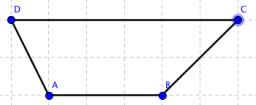# Trapezoid 2520

Trapezoid with sides a = 10, b = 20, c = 25, d = 15. Calculate all internal angles.

α =  96.3791 °
β =  131.8103 °
γ =  48.1897 °
δ =  83.6209 °

### Step-by-step explanation:Did you find an error or inaccuracy? Feel free to write us. Thank you!

Tips to related online calculators
Do you have a linear equation or system of equations and looking for its solution? Or do you have a quadratic equation?